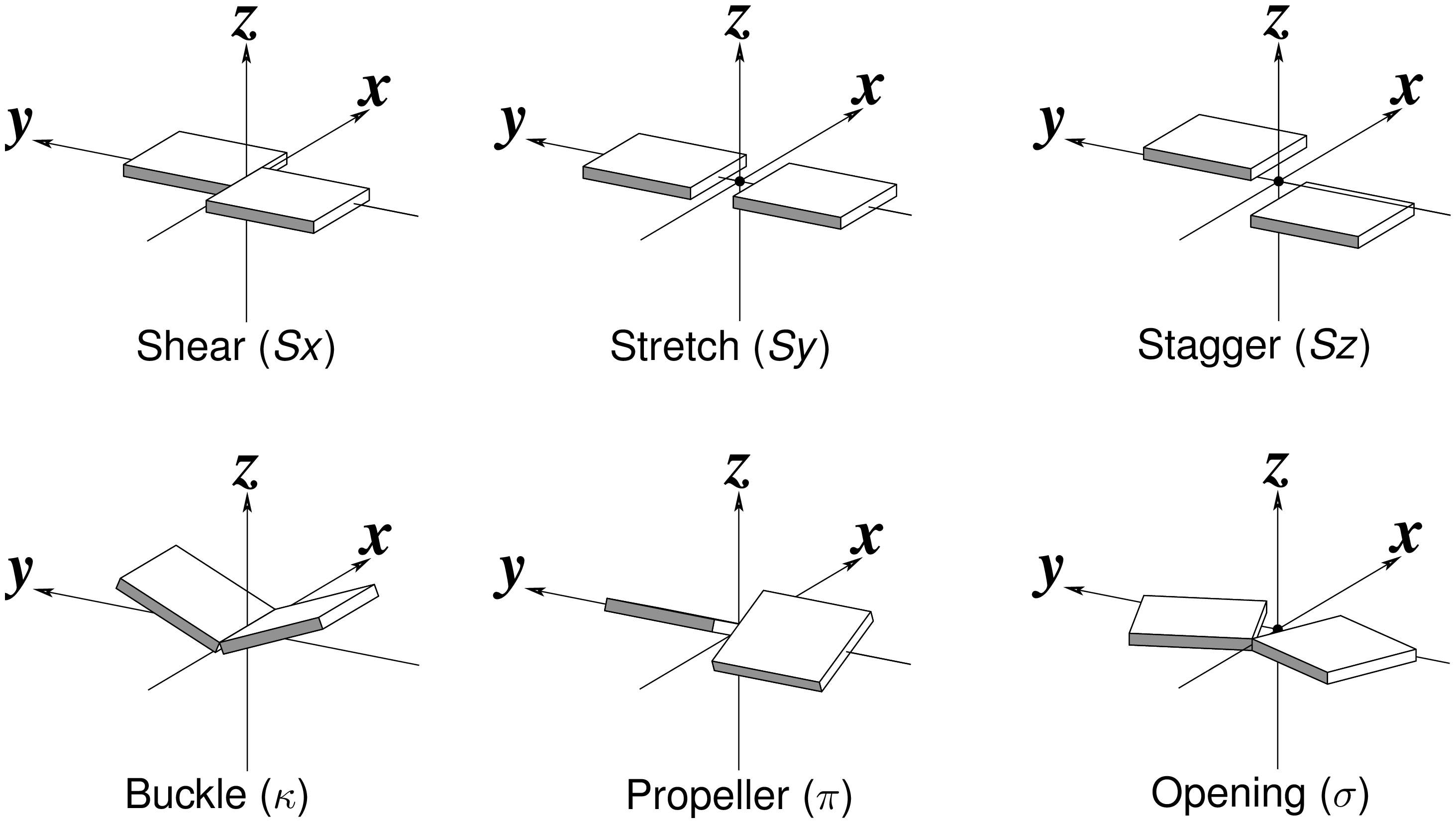# Simple base-pair parameters

Recently, I read with great interest an article titled A context-sensitive guide to RNA & DNA base-pair & base-stack geometry by Dr. Jane Richardson, published in CCN (Computational Crystallography Newsletter, 2015, 5, 42—49). Highlighted in the article are Buckle and Propeller twist (see bottom left of the figure below), two of the angular parameters that characterize base-pair (bp) non-planarity. Particularly, I was intrigued by the “Notes on measures and figures” at the end:

Base normals were constructed in Mage (Richardson 2001) and twist torsions and buckle angles were measured from them; propeller-twists were measured as dihedral angles around an axis between N1/9 atoms.The Richardson CCN article prompted me to think more on intuitive description of bp geometry that can be easily grasped by experimentalist, especially X-ray crystallographers or cryo-EM practitioners. Without worrying about model building as with the six rigid-body parameters, it is straightforward to come up with a new set of four ‘simple’ parameters (Shear, Stretch, Buckle and Propeller) with the following characteristics:

• Each parameter can be positive or negative. For type M–N pairs (as in the canonical cases), Shear and Buckle reverse their signs when the two bases are swapped (i.e. counted as N–M instead of M–N). In all other cases, the signs of the parameters remain unchanged. See the DSSR paper for the definition of M+N vs M–N type of pairs.
• Intuitive results for non-canonical pairs, even when Opening is ~180º.
• Consistent definition between Shear/Buckle (x-axis) vs Stretch/Propeller (y-axis).
• As in 3DNA and DSSR, Buckle^2 + Propeller^2 = interBase_angle^2. Either Buckle or Propeller can render the two base planes of a pair non-parallel. Combined together, they introduce a non-zero inter-base angle. By definition, each parameter should not be larger than the overall inter-base angle.

With the cartoon-block representation introduced in DSSR, base-stacking interactions and bp deformations (especially Buckle and Propeller) are immediately obvious. Two example are illustrated in the figure below: one is the classic Dickerson B-DNA dodecamer (355d, DSSR output), and the other is the parallel double-stranded helix of poly(A) RNA (4jrd, DSSR output).

A portion of DSSR output for the B-DNA duplex 355d is shown below. Note that the first bp (at the bottom left in the figure above) has a Propeller of –17º (and a Buckle of +7º). As beautifully explained by Calladine et al. in their book Understanding DNA, The Molecule & How It Works, Watson-Crick pairs prefer to have negative Propeller in right-handed DNA double helices to improve same-strand base-stacking interactions. The average value of Propeller in A- and B-DNA crystal structures is around –11º (see Table 3 of the Olson et al. standard base reference frame paper).

```     nt1            nt2           bp  name        Saenger    LW  DSSR
1 A.DC1          B.DG24         C-G WC          19-XIX    cWW  cW-W
[-105.9(anti) ~C2'-endo lambda=53.5] [-141.3(anti) ~C3'-endo lambda=52.7]
d(C1'-C1')=10.71 d(N1-N9)=8.96 d(C6-C8)=9.88 tor(C1'-N1-N9-C1')=-21.4
H-bonds: "O2(carbonyl)-N2(amino)[2.83],N3-N1(imino)[2.90],N4(amino)-O6(carbonyl)[2.98]"
interBase-angle=19  Simple-bpParams: Shear=0.28 Stretch=-0.13 Buckle=7.3 Propeller=-17.2
bp-pars: [0.28    -0.14   0.07    6.93    -17.31  -0.61]
2 A.DG2          B.DC23         G-C WC          19-XIX    cWW  cW-W
[-85.4(anti) ~C2'-endo lambda=53.4] [-150.3(anti) ~C3'-endo lambda=55.4]
d(C1'-C1')=10.61 d(N1-N9)=8.92 d(C6-C8)=9.83 tor(C1'-N1-N9-C1')=-21.7
H-bonds: "O6(carbonyl)-N4(amino)[2.91],N1(imino)-N3[2.88],N2(amino)-O2(carbonyl)[2.88]"
interBase-angle=17  Simple-bpParams: Shear=-0.24 Stretch=-0.18 Buckle=9.0 Propeller=-14.5
bp-pars: [-0.24   -0.18   0.49    9.34    -14.30  -2.08]
```

A portion of DSSR output for the parallel A-DNA duplex 4jrd is shown below. Note that the values of ‘simple’ Propeller are positive for both bps #7 and #8. In contrast, the rigid-body bp parameters have their signs flipped over when Opening is switched from –179.56º for bp#7 to +179.23º for bp#8. This sign ‘ambiguity’ around 180º Opening could be confusing. Yet, all the six bp parameters must be kept as they are for rigorous rebuilding, especially within a larger context than a bp per se. From the very beginning, 3DNA has adopted the convention of keeping angular parameters in the range of [–180º, +180º] instead of [0, 360º], allowing left-handed Z-DNA to have negative twist.

```   7 A.A8           B.A7           A+A --          02-II     tHH  tM+M
[-175.8(anti) ~C3'-endo lambda=10.2] [-172.7(anti) ~C3'-endo lambda=12.6]
d(C1'-C1')=11.15 d(N1-N9)=8.29 d(C6-C8)=6.31 tor(C1'-N1-N9-C1')=160.1
H-bonds: "OP2-N6(amino)[2.97],N7-N6(amino)[2.97],N6(amino)-OP2[2.92],N6(amino)-N7[2.91]"
interBase-angle=14  Simple-bpParams: Shear=-7.88 Stretch=0.66 Buckle=-7.8 Propeller=11.9
bp-pars: [-6.00   5.15    -0.02   0.63    14.22   -179.56]
8 A.A9           B.A8           A+A --          02-II     tHH  tM+M
[-177.4(anti) ~C3'-endo lambda=12.4] [-175.8(anti) ~C3'-endo lambda=10.3]
d(C1'-C1')=11.01 d(N1-N9)=8.15 d(C6-C8)=6.18 tor(C1'-N1-N9-C1')=158.5
H-bonds: "OP2-N6(amino)[2.93],N7-N6(amino)[2.88],N6(amino)-OP2[2.97],N6(amino)-N7[2.92]"
interBase-angle=15  Simple-bpParams: Shear=-7.91 Stretch=0.56 Buckle=-7.0 Propeller=13.7
bp-pars: [6.11    -5.06   -0.05   -2.26   -15.22  179.23]
```## Comment

 Name Remember Email Website Message Textile helpThank you for printing this article from http://home.x3dna.org/. Please do not forget to visit back for more 3DNA-related information. — Xiang-Jun Lu